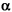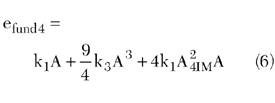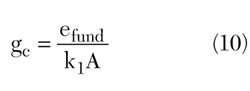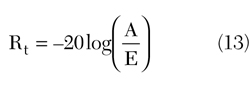This article continues the trend of previous publications,1,2 to simplify the prediction of RF performance of systems designed for wireless digital communications. The first shows the correlation between sidelobes and intermodulation (IM) products. Sidelobes are the result of the spectrum re-growth when a digitally modulated signal is applied to the input of a nonlinear amplifier. IM products are the result of the creation of additional products when sinusoidal (CW) signals are applied to the input of the same amplifier. Clearly it is much easier to predict the behavior of sinusoidal signals. That is why the initial discussion in this article focuses on the reduction of IM products appearing with a CW excitation. The understanding of an amplifier's adjacent channel power (ACP) reduction with generic digital excitation is derived based on the CW results.There are several general techniques of nonlinearity effects reduction. They are divided into two major groups. The first one (called feed forward linearization) is geared toward compensation of nonlinear effects after their appearance. The second group focuses on preventing those effects from appearing. It is called "predistortion." According to this technique, the input signal is distorted in a specific way allowing the output signal to be distortion-free (that is, allowing the circuit to reduce the output's ACP value).

The method of ACP improvement based on predistortion is straightforward and well known. The idea is to create signals at the adjacent channel frequencies (sidelobes) with the same amplitude but opposite phase to the ones produced by the amplifier itself. What is new in this article is the approach to the solution. Instead of concentrating on the compensation of nonlinearity expressed as input/output transfer characteristics,3 it focuses on the root of the problem, that is, on the elimination of spectrum sidelobes. It can be proved that the suggested approach produces the expected transfer characteristic. However, focusing on the creation of spectrum sidelobes in anti-phase to the ones produced by the amplifier nonlinearity helps to come up with an easy and low cost circuit capable of compensation close to the theoretical limit.

In this article, the theory of a spectrum re-growth creation is introduced. It is first explained with the example of a two-tone excitation; the compensation of sidelobes (results of the spectrum re-growth due to a digitally modulated signal) is then introduced and a method for its compensation is given. The simulations and measurements confirm the approach.

#### Basic Theory

Any nonlinear system can be described by the equation

eout = k1ein + k2e2in + k3e3in + ... (1)

where

ein = input signal

eout = output signal

ki = complex coefficients

One of the easiest ways to evaluate a nonlinear system is to apply sinusoidal signals to its input. The most commonly used combination is when two signals of the same amplitude but different frequencies (two-tone signals) are applied. It is described by the relationwhere

A = amplitude of each of the input signal tones,= frequencies of each of the input signal tones

Using straightforward calculations, the amplitude of the output signal at each fundamental frequency is given by4Any nonlinear system with multitone CW signals at its input produces IM, appearing as a result of interaction between the tones. For two-tone input signals, IM products are appearing at the frequencies (2±) and (2±). Their amplitude is4Equations 3 and 4 demonstrate the effect of nonlinearity on the system's output. For an ideal linear system, the coefficient k3 is equal to 0, and the system would just amplify the input signal without creating any intermodulation products. For a nonlinear system (k3 is not equal to 0), the value of the output signal would change, compared to the linear system, and IM products would appear. Depending on the sign of k3, the value of efund from Equation 3 can increase (gain expansion) or decrease (gain compression). It is relatively easy to compensate the gain variations of the system. A more serious problem occurs with the creation of IM products, since they may occur at the frequencies of adjacent channels. However, not all IM products create problems with a system's design since the ones at frequencies far away from the fundamental can be easily filtered out. The products which cannot be eliminated by filtering are at frequencies of (2-) and (2-). They could be eliminated by adding tones of the same amplitude and opposite polarities to the output of a nonlinear system. A natural way to implement the addition is to apply an additional two CW tones at the frequencies of IM products to the input of the nonlinear system.where

A4IM = amplitude of each of additional input signal tones, applied to eliminate the IM products

The output amplitude at the fundamental frequencies is4At the (2-) and (2-) frequencies the amplitude of a resulting signal isIn Equation 7, the first two components result from intermodulation, the third component is the result of a triple beat, and the last component is the linear gain of one of the tones with amplitude A4IM.

eIM4 must be equal to 0, which happens whenwhere

r2ab/a = relative value of the third-order IM products

It is found from the relation4gc = relative value of gain compression4From Equation 8, the value of A4IM (that is, the amplitude of each additional input signal applied to compensate output IM products) is found. It can be found for any combination of nonlinearity coefficients ki regardless of whether they are real or complex. In the case of complex coefficients, Equation 8 is modified toThis result shows that the two-tone IM products are completely and unconditionally eliminated by applying two additional tones, the amplitude and phase of which are found from Equation 11. It is straightforward to demonstrate that the fifth-order IM products (that is, products proportional to the coefficient k5 in Equation 1) are not going to produce any significant influence on this result.Unfortunately, this easy and unconditional compensation becomes impossible in some cases when more than two CW signals are applied to the input of a nonlinear system. The reason is that additional IM products may occur at the frequencies of previously created IM products, as demonstrated in Figure 1 , representing the output spectrum of a nonlinear system.

Initially, the two-tone input was producing two tones of amplitude A at fundamental frequenciesandand two IM products of amplitude C at frequencies (2-) and (2-). If two more tones at fundamental frequenciesandare applied to the input, they produce two tones with amplitude B and two of their own IM products with amplitude D. Ifandhappen to be related toandby the relation 2(-)=(-), then one IM product with amplitude D is going to occur at the frequency 2-, that is, the frequency of one of the initial IM products C. It means that the resulting amplitude of the signal at that frequency is going to be equal to a combination of C and D.

At first glance it seems that this situation (more than one IM product landing on the same frequency) does not create a big problem for compensation. It seems that it is still possible to achieve a complete and unconditional compensation of IM products with some amplitude (and possibly phase) adjustments. The complete compensation would be indeed possible if compensation of IM products appearing only at a single frequency 2-was of interest. However, multiple CW signals create multiple unwanted IM products at a number of frequencies (such as E and E1 in Figure 2 ).At this point, it is still not obvious that multiple unwanted IM products at a number of frequencies create a problem. It seems that multiple unwanted IM products could be compensated by creating a number of input signals producing outputs with the same amplitude but opposite phase to all IM products.

The nature of the problem becomes obvious when large compensating signals are considered. Large compensating signals interact with the original CW signal. As a result of this interaction, IM products are created, which are impossible to compensate. In the previous example, the tone with amplitude B1 interacts with the compensating tone of amplitude E. The interaction creates IM products. Those newly created IM products can occur at the same frequencies as the IM products that were going to be compensated in the first place. If the tone B1 is at the frequency (-/2), then the newly created IM product of interaction between B1 and E occurs at the frequency of product with amplitude E1.

This constitutes a limitation of predistorter compensation. In this case, that means the compensation could not be complete and unconditional. The compensation could not become better than the value of the IM products resulting from interaction between the original CW and compensating signals (if those IM products fall at the frequencies of the compensating signals).

In a realistic case, there will be a number of original CW tones, as well as a number of compensating tones. Now focus on just two tones: the main and compensating tones. The amplitude of the IM product created by those two tones is found from the relationwhere

A = amplitude of a main tone

E = amplitude of a compensating tone

The amplitude of the original IM products (that is, the products the compensating signals are designed to get rid of) is found from Equation 4.

Since E (the amplitude of the compensating tone) is significantly smaller than A (the amplitude of the main tone), the amplitude of the IM product given by Equation 12 is much smaller than the amplitude of the IM product from Equation 4. So the improvement due to the compensating signals is still obvious, only it cannot become better than the value given by Equation 12. This improvement is expressed in decibels by the formulaFor a 1 dB compressed amplifier, the difference between A and E is approximately 28 dB.5 The more the amplifier is compressed, the smaller this difference becomes. The above calculation suggests that, even in the simplest case, the effects of nonlinearity in a 1 dB compressed amplifier cannot be improved by more than 28 dB.

In the realistic case of a number of original CW and compensating tones, some of the IM products are going to occur at the same frequencies. Assuming that they are of the same phase, the amplitude of their sum is going to be higher than predicted by Equation 12. It will further reduce the limitations for compensation. The formula for the resulting improvement by compensating signals is given bywhere

N = the number of IM products landing at the same frequency

If, for instance, 100 IM products land at the same frequency, then 10log(N) = 20 dB. It means that the linearity of the same amplifier could possibly be improved, not by 28 dB, but only by 8 dB.

In a discrete spectrum, N is limited by the spacing between tones. In reality, the IM products created by two-tone signals are produced at frequencies determined by a simple rule - the difference in frequencies between each of two tones is the same as the difference in frequencies between the resulting IM product and the second tone. In the example of multiple CW signals, the tone of amplitude A at frequencywith the tone of the same amplitude A at frequency a produces an intermodulation product at frequency 2-. The difference in frequencies between each of the tones is-. The difference in frequencies between the IM product and the second tone is (2-) -=-, that is, the same as between tones. Thus, in a discrete spectrum, the IM products occur at the same frequency only if the appropriate tones are available. The availability of the tones is determined by this rule - the frequency of the second tone must be equal to one half of the difference in frequencies between the first tone and the IM product itself. Using the same example, the tone with amplitude A at frequencyinteracting with the tone of amplitude B at frequencycan create an IM product at frequency 2-ifhappens to be meeting the rule. That is,has to be equal to 1/2((2-) -) = (-)/2.

The spectrum created by digitally modulated signals is a continuous spectrum. Technically, it is created by different rules than a discrete spectrum. The input signal is the product of a function representing a digital data stream (a type of sin (x)/x function) by a function representing some type of a filtering (usually root-raised-cosine filter). The output sidelobes are appearing due to the spreading of the spectrum by higher order components (when the input signal is substituted into Equation 1). However, for a continuous spectrum, it is not easy to get readily explainable numerical results. At the same time, a continuous spectrum can be thought of as a number of discrete harmonics spaced infinitely close to each other.1 It means that the results of CW evaluations can be applied to estimate the effects of a continuous spectrum. Since in a CW presentation of a continuous spectrum, the tones are considered to be infinitely close to each other and all the required tones for the occurrence of IM products at the same frequency are present.

That is, the results of Equations 12 and 14 can be applied to digitally modulated signals. In Equation 14, though, the value of "N" has to be supplied. To approximate it for a continuous spectrum, the value of resolution at which each level of power at an adjacent channel frequency is measured can be used. A typical ratio of bandwidth-to-resolution is approximately 1 to 0.5 percent. It makes the number of effective tones contributing to the creation of additional IM products to be between 100 and 200. By introducing N = 100 to 200 in Equation 14, Pr is found to be approximately 5 to 8 dB. In other words, the result predicted by Equation 12 is going to be worsened by 20 to 23 dB.

The number obtained from Equation 14 sets an upper limit of RF predistortion. It indicates that, in principle, a predistorter cannot correct certain heavily compressed nonlinear systems. That is, if the nonlinear system produces sidelobes exceeding 23 dB below its main lobe, no RF predistorter can correct them.

The lower limit of predistortion is determined by the spectrum of digitally modulated input (that is, linear) signal. Depending on the characteristics of root-raised-cosine filters, it is approximately 60 to 70 dB.

The results prove that the RF predistortion can improve the ACPR of digitally modulated signals on mildly nonlinear (that is, well below the 1 dB compression point) systems by 10 to 15 dB. To implement this improvement one has to apply a compensating signal to the input of a nonlinear system. This signal should be of small (limited) bandwidth with the same amplitude and opposite polarity to the sidelobes of an initial signal. The block diagram depicting this concept is given in Figure 3 .The upper branch provides a path for an undistorted input signal to be applied to the nonlinear system. The lower branch consists of three blocks. The first one is a generator of distortions. Its function is to provide distortions of a sufficient amplitude and limited bandwidth. Any nonlinear device with a high degree of nonlinearity should do the job. The easiest (however, not always the least expensive and, very often, not the optimum) way to implement the generator of distortions is to use a device of the same technology as the nonlinear system itself. The second and third blocks are a variable attenuator and a variable phase shifter, respectively. Their function is to adjust the output of the generator of distortions in a way that distortions show up at the systems' output with the same amplitude and opposite polarity to the sidelobes. Finally, the variable gain block is used to adjust the output power to the required level. It is needed to compensate for the partial input carrier cancellation due to the operation of the predistorter. Simulations based on the previously described block diagram confirm the results of this section.

#### Simulation

Simulations were conducted on a platform created within an Agilent ADS software package. This platform consisted of a signal source and an amplifier. The function of the signal source is to create a signal, corresponding to a specific modulation scheme. An NADC source was chosen for simulations since this standard does not require additional licenses for simulation. The parameters calculated by the software are the peak and average powers in the time domain and the spectrum in the frequency domain. The detailed descriptions of the simulation platform as well as the simulation files are available upon request. An example of the simulation results is given in Figure 4 ; the results of the simulation are given in Table 1 .These results agree with the predictions of the preceding section. The first row represents the improvements by the predistorter on a 1 dB compressed amplifier. As it was shown in reference 1, the NADC amplifier's averaged ACP coincides with the ratio between the main tone and the IM product for a two-tone exited amplifier. The ratio between the main tone and the IM product for a 1 dB compressed amplifier is 28 dB.1,5 The same ratio for a 0.5 dB compressed amplifier is approximately 35 dB (the second row). The ACPR improvement column coincides with the predictions by Equation 14 for N = 100 to 200. The third row represents a perfectly linear amplifier that is an amplifier below the lower limit of predistortion. The results are confirmed by measurements.

 Table 1 Simulation Results Small Signal Gain (dB) Large Signal Gain (dB) Input Power (dBm) Gain Compression (dBm) ACPR Improvement (dB) Prediction by CW Method (dB) 36 35.0 -5.7 30 7 5 36 35.5 -8.9 30 12 12 36 36.0 -30.0 30 0 N/A

#### MeasurementsFigure 5 demonstrates that, for a two-tone excited amplifier, the achievable improvement is high. The initial marker was set to the IM product produced by the nonlinearity of the amplifier. The second marker shows the difference between the uncorrected two-tone output and the output with RF predistortion. A compensation of more than 29 dB is difficult to achieve, since at such low power levels, the influence of higher order products becomes noticeable. This influence is realized by the addition of IM products generated by higher tones to the frequency of the initial IM product (no contradiction to the remarks to Equation 11).

However, as predicted, the results are much worse for digitally modulated signals. Figures 6 and 7 represent the output of an LDMOS-based power amplifier. The output power in both cases was kept at 45 dBm.The difference between them is in the excitation of the amplifier. The first graph shows the improvement by the predistorter with the uplink WCDMA signal applied to its input. The peak-to-average power ratio of that signal is 3.5 dB. The predistorter improves the sidelobes (depending on the frequency offset) by 6 to 10 dB. The second graph demonstrates the improvement by the predistorter with the downlink WCDMA signal applied to its input. The peak-to-average power ratio of the downlink signal is already 11 dB, that is, the amplifier is compressed much heavier than in the previous case. That heavier compression allows an ACPR improvement of only a few decibels.

#### Conclusion

In this article, a theoretical concept of RF predistortion based on the spectrum re-growth model was introduced. It was stated that the idea behind RF predistortion is in compensating sidelobes created by the amplifier's nonlinearity. The method to achieve compensation is to apply a compensating signal with the same amplitude and opposite phase to the initial sidelobes.It was shown that for individual IM products created by CW signals, any degree of compensation could be achieved. The problem with the achievable degree of compensation occurs only when the amplifier's nonlinearity creates sidelobes (vs. individual IM products). The reason is in the interaction of the compensating signal with the initial one. Because of the relatively wide bandwidth of the sidelobes to be compensated and the relatively large amplitude of the compensating signal, the interaction leads to the creation of its own sidelobes. Those newly created sidelobes are impossible to compensate by RF predistortion. Their value is in direct proportion to the amplitude and bandwidth of a compensating signal.

These newly created sidelobes set the limit on the achieved compensation by the RF predistorter. For all practical purposes, this type of predistorter can be used only on amplifiers with the combined level of nonlinearity not exceeding the 1 dB compression point. The achievable ACPR improvement of digitally modulated signals on mildly nonlinear (that is, well below 1 dB compression point) amplifiers by RF predistortion is 10 to 15 dB.

Simulations and measurements confirm the predictions. Simulations were conducted using a TDMA (NADC) waveform, to exclude an uncertainty of multi-channel excitation. Measurements were done on the same LDMOS amplifier with three types of input signals, that is, a CW signal, a single channel WCDMA signal and a multi-channel WCDMA signal. It was expected to get a near perfect cancellation in the case of the CW signal, reasonable cancellation for a single channel WCDMA signal and almost no cancellation on a multi-channel WCDMA signal. Simulations predicted an ACPR improvement of 7 to 12 dB, measurements of a single channel WCDMA signal provide 6 to 10 dB improvement and measurements of a multi-channel WCDMA signal show only a few decibels of improvement. n

#### References

1. B. Aleiner, "Correlation Between P1dB and ACP in TDMA Power Amplifiers," Applied Microwave & Wireless , No. 3, March 1999, pp. 82-94.
2. B. Aleiner and O. Zakhalyavko, "The Influence of LO Harmonics on Receiver Performance in the Modulation Schemes Used for Wireless Communications," Microwave Journal , Vol. 44, No. 9, September 2001, pp. 138-146.
3. K. Morris and P. Kenington, "Power Amplifier Linearization Using Predistortion Techniques," IEE Colloquium on RF and Microwave Components for Communications Systems , April 1997, Bradford, UK.
4. K.A. Simons, "The Decibel Relationships Between Amplifier Distortion Products," Proceedings of the IEEE , Vol. 58, No. 7, July 1970.
5. S.M. Perlow, "Third-order Distortion in Amplifiers and Mixers," RCA Review , Vol. 37, June 1976.Boris Aleiner earned his MSEE degree from Leningrad Polytechnic University, Leningrad, Russia. He began his career as a microwave engineer in Leningrad in 1974, and came to the US in 1982, where he worked for several RF components companies. In 1990, he began working in the wireless sector for Bell Laboratories, and in 1992 became a member of the company's technical staff. He joined Motorola in 1998. Currently an independent consultant, he has published five papers on the subject of RF wireless components/subsystems and holds several US patents on the same subject.